# Transformer

Transformer - U1 = 230 V, N1 = 300, N2 = 1,200, I1 = 4 A. Calculate the transformation ratio, voltage and current in the secondary coil.

k =  4
U2 =  920 V
I2 =  1 A

### Step-by-step explanation: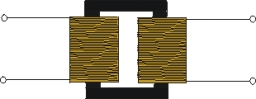Did you find an error or inaccuracy? Feel free to write us. Thank you!## Related math problems and questions:

• Coils of transformerThe primary coil of the transformer has 400 turns, a current of 1.5 A passes through it and is connected to a voltage of 220 V. For the secondary coil, find the voltage, current, and a number of turns if the transformation ratio k = 0.1.
• The output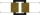The output voltage of the transformer is 880 V. The secondary coil has 1200 turns. Find the voltage to which the primary coil is connected and how many turns it has if a current of 1 A flows through it. Transformation ratio k = 4. What current flows throu
• Coils of transformerThe primary coil of the transformer has 1100 turns and is connected to a voltage of 220V. How many turns does the secondary coil have when the voltage on it is 55 V? Find the transformation ratio and decide what kind of transformation.
• Coils of transformerWhat voltage is generated on the secondary coil of the transformer, which has 1500 turns, if the primary coil is connected to a voltage of 120 V and has 500 turns? What is the transformation ratio and what is the transformation about?
• The transformerThe primary coil of the transformer has 1100 turns and is connected to a voltage of 220 V. How many turns does the secondary coil have when it has a voltage of 55 V? Determine the transformation ratio and decide what the transformation is.
• ResistanceDetermine the resistance of the bulb with the current 200 mA and is in a regular lamp (230V).
• Electric input powerSolve problems related to electric power: a) U = 120 V, I = 0.5 A, P =? b) P = 200 W, U = 230 V, I =? c) I = 5 A, P = 2200W, U =?
• Cooker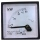A current of 2A passes through the immersion cooker at a voltage of 230V. What work do the electric field forces in 2 minutes?
• Mains voltageHow much electric current flows through the appliance with a resistance of 40kΩ, which is connected to the mains voltage (230 V)?
• TransformerSolve the textbook problems - transformer: a) N1 = 40, N2 = 80, U2 = 80 V, U1 =? b) N1 = 400, U1 = 200 V, U2 = 50 V, N2 =?
• Electric workCalculate the work done by the electric forces passing the current of 0.2 A through the bulb in 10 minutes if the bulb is connected to a 230 V power supply.
• Energy consumption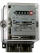The device is connected to 230V and draws 3.5A current. Power consumption is 1932kJ. How many minutes has this device been in operation?
• Kirchhoff's lawTwo resistors with 100 Ω and 300 Ω resistors are connected in series. A current of 1.8 A passes through the first 100Ω resistor. What current flows through the second resistor?
• Resistor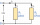Resistor 1 with a resistance of 100 Ohms and resistor 2 with a resistance of 400 Ohms are connected side by side in the circuit. There is a voltage of 80V between the resistor terminals. a) Draw a circuit diagram and write the entered quantities in it b)
• Two resistorsTwo resistors 20 Ω and 60 Ω are connected in series and an external voltage of 400 V is connected to them. What are the electrical voltages on the respective resistors? Please comment!
• Coil as a girl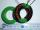The electrical resistance of the copper wire coil is 2.0 ohms. What current runs through the coil when the voltage between the terminals is 3.0 V?
• Current in the conductorCalculate the current in the conductor (in mA) if it is connected to a 4.5 V voltage source and its resistance is 20 (ohm).